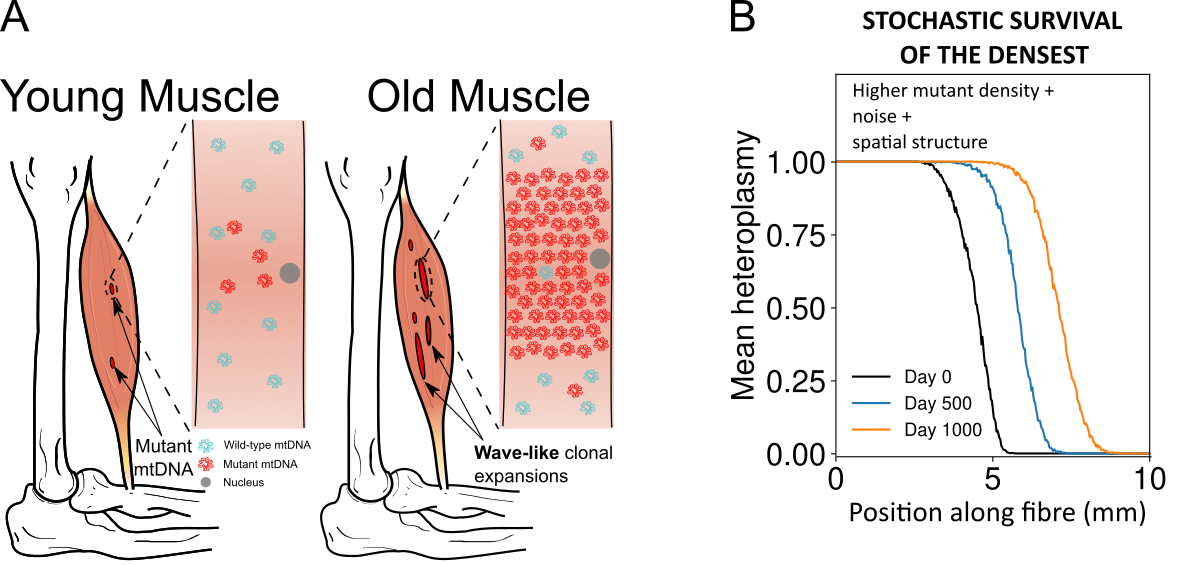## Tuesday 7 February 2023

### Community detection in graphons

Graphs are one way to represent data sets as they arise in the social sciences, biology, and many other research domains. In these graphs, the nodes represent entities (e.g., people) and edges represent connections between them (e.g., friendships). For the analysis of graphs, a vast selection of mathematical and computational techniques has been developed. For example, Google uses eigenvectors to quantify the importance of webpages in a graph that represents the word-wide web.

Community detection

One field of interest in the analysis of graphs is the detection of so-called communities. These are groups of nodes that are strongly connected internally but only sparsely to nodes in other groups. A common example in the social sciences are friendship groups. Community-detection methods can be used to cluster large graph-structured data sets and so provide insights into many complex systems. For example, one can use the community structure to investigate how brain function is organised. In these biological graphs, the different communities represent different parts of the brain, each of which fulfilling different functions. Many algorithms to identify communities exist, the most popular of which is modularity maximisation.

Graphons

As the scale of these data sets increase, the graphs that represent them get larger, too. This makes the development of computationally-efficient tools for their analysis a pressing issue. One way to investigate these large, finite objects is to abstract them with idealized infinite objects. In this context, graphons have emerged as one way of looking of infinite-sized graphs.

One way to motivate graphons is to look at graphs that grow in size (i.e., the number of nodes) by iteratively adding nodes and edges. In Fig. 1, we show graphs of varying size in a so-called pixel image. Each pixel image visualises the edges in a graph with n nodes. The n^2 pixels in each image represent a single pair of nodes and are RED if an edge is connecting the pair of nodes and WHITE if not. With growing network size n, we observe that the pixels get smaller, making the discrete image resemble a continuous density plot. The graphon, as shown on the very right, is the continuous limiting object of such growing graphs as n goes to infinity. This intuitive notion of convergence can be concretised and proven by looking at subgraph counts.

Defining community detection for graphons

In our paper, which was just published in SIAM Applied Mathematics "Modularity maximization for graphons" (free version here) joint with Michael Schaub, we explore how one can define and compute these communities for graphons. To achieve this, we define a modularity function for graphons, which is an infinite-sized limit for the well-established modularity function for graphons. The modularity function for graphs is defined as a function of a double-sum over all pairs of edges. The core idea is, that in the infinite-size limit (i.e, for graphons) this double sum approaches a double integral, which may simplify the computational burden of community detection.

In our paper, we explore a selection of synthetic graphons and compute their community structure. Surprisingly (at least for us), the continuous form of graphons allows us to analytically compute the optimal community structure for some of these --- something that is usually not possible for graphs. Lastly, we outline a computational pipeline that would allow us to detect community structure in a privacy-preserving way (see Fig. 2). Florian and Nick.